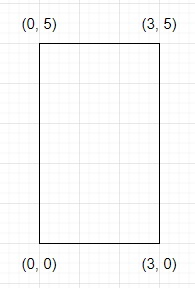# Program to find perimeter of a polygon in Python

Suppose we have a list of ordered points represents a simple polygon endpoint on a 2D plane. We have to find the perimeter of this polygon.

So, if the input is like points = [(0, 0), (0,5), (3, 5), (3,0)], then the output will be 16 becausetwo sides are of length 3 and two sides are of length 5, so 2*5 + 2*3 = 16.

To solve this, we will follow these steps −

• Define a function getInfo() . This will take x1, y1, x2, y2
• return square root of ((x1-x2)*(x1-x2)+(y1-y2)*(y1-y2)) which is Euclidean distance
• between (x1, y1) and (x2, y2)
• From the main method, do the following
• N := size of points
• (firstx, firsty) := points
• (prevx, prevy) := (firstx, firsty)
• res := 0
• for i in range 1 to N-1, do
• (nextx, nexty) := points[i]
• res := res + getInfo(prevx, prevy, nextx, nexty)
• prevx := nextx
• prevy := nexty
• res := res + getInfo(prevx, prevy, firstx, firsty)
• return res

## Example

Let us see the following implementation to get better understanding −

from math import sqrt
def getInfo(x1, y1, x2, y2):
return sqrt((x1-x2)*(x1-x2)+(y1-y2)*(y1-y2))

def solve(points):
N = len(points)
firstx, firsty = points
prevx, prevy = firstx, firsty
res = 0

for i in range(1, N):
nextx, nexty = points[i]
res = res + getInfo(prevx,prevy,nextx,nexty)
prevx = nextx
prevy = nexty
res = res + getInfo(prevx,prevy,firstx,firsty)
return res

points = [(0, 0), (0,5), (3, 5), (3,0)]
print(solve(points))

## Input

[(0, 0), (0,5), (3, 5), (3,0)]

## Output

16.0